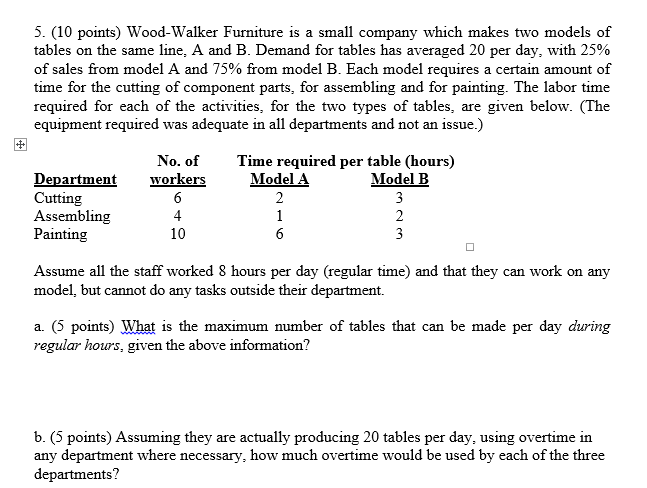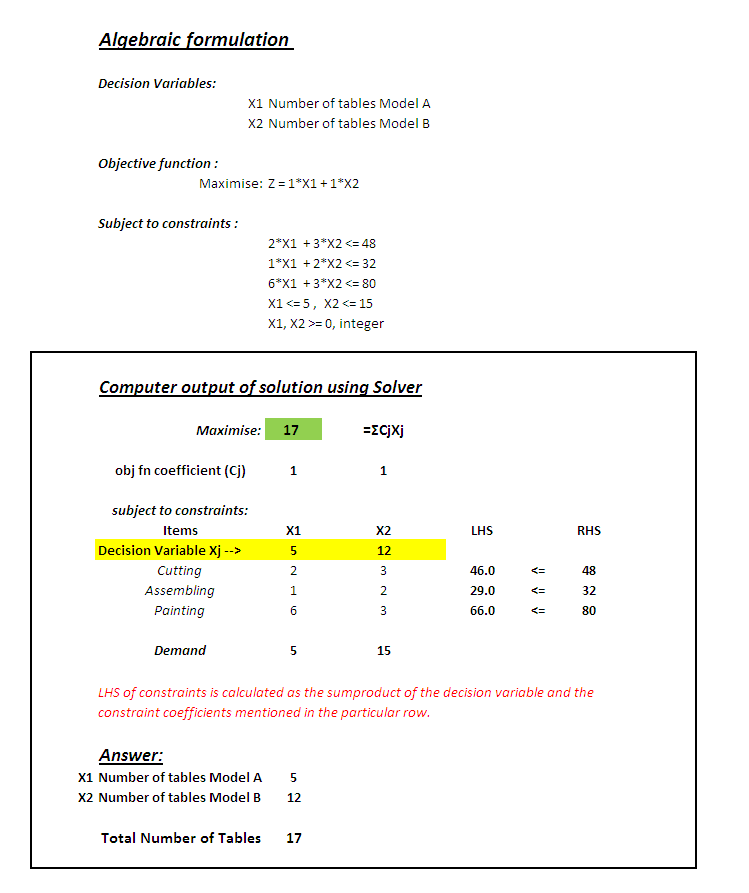# Question & Answer: Wood-Walker Furniture is a small company which makes two models of tables on the same line, A and B……Wood-Walker Furniture is a small company which makes two models of tables on the same line, A and B. Demand for tables has averaged 20 per day, with 25% of sales from model A and 75% from model B. Each model requires a certain amount of times for the cutting of component parts, for assembling and for painting. The labor time required for each of the activities, for the two types of tables, are given below. (The equipment required was adequate in all departments and not an issue.) Assume all the staff worked 8 hours per day (regular time) and that they can work on any model, but cannot do any tasks outside their department. What is the maximum number of tables that can be made per day during regular hours, given the above information? Assuming they are actually producing 20 tables per day, using overtime in any department where necessary, how much overtime would be used by each of the three departments?

Demand

Demand for Table A = 25%*20 = 5

Don't use plagiarized sources. Get Your Custom Essay on
Question & Answer: Wood-Walker Furniture is a small company which makes two models of tables on the same line, A and B……
GET AN ESSAY WRITTEN FOR YOU FROM AS LOW AS \$13/PAGE

Demand for Table B = 75%*20 = 15

Available worker time (hours)

Cutting     = 6*8                 = 48

Assembling    = 4*8          = 32

Painting    = 10*8              = 80

We will solve part (a) of the problem using linear programming

Decision Variables

Number of Tables A produced X1

Number of Tables B produced X2

Constraints

Time required per table in each department

Objective function

Maximise total number of tables produced per day subject to the demandb. Overtime used by each time is simply calculated by subtracting the worker time available from the time required for making 20 tables as below

 WTR WTA Items Time required for Model A Time required for Model B Worker Time Required = 5*A + 15*B Worker Time Available Overtime required (hours) = WTR-WTA Cutting 2 3 55 48 7.0 Assembling 1 2 35 32 3.0 Painting 6 3 75 80 0.0